# MODE

Use the MODE function in Excel to find the most frequently occurring number in a list of numbers. Use MODE.MULT to find multiple modes.

1. The MODE function below returns the most frequently occurring number (8).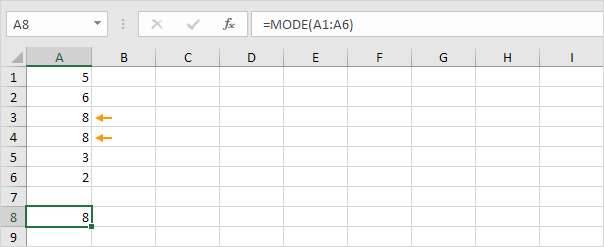2. The new MODE.SNGL function (SNGL stands for single) produces the exact same result.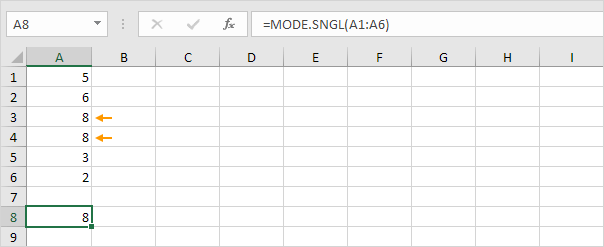3. Change the value in cell A2 to 5. In this example, there are multiple modes (5 and 8). MODE and MODE.SNGL always return a single mode.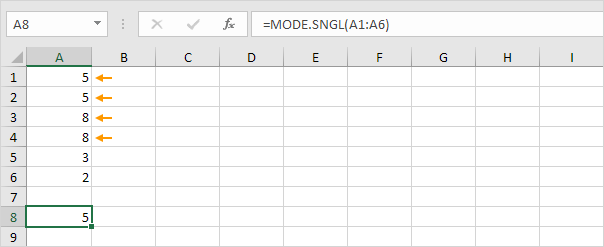4. If you have Excel 365 or Excel 2021, simply use the MODE.MULT function to find multiple modes.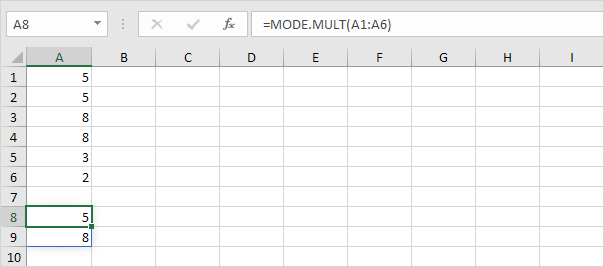Note: the MODE.MULT function, entered into cell A8, fills multiple cells. Wow! This behavior in Excel 365/2021 is called spilling.

If you don't have Excel 365 or Excel 2021, execute the following steps to find multiple modes.

5. Select multiple cells.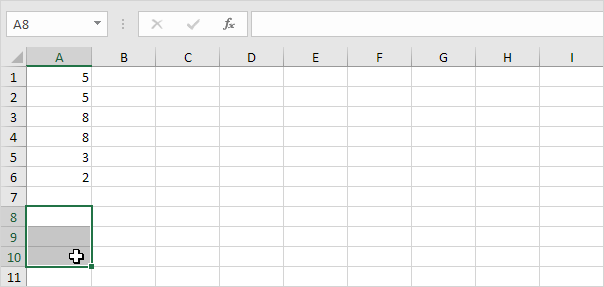6. Enter the MODE.MULT function.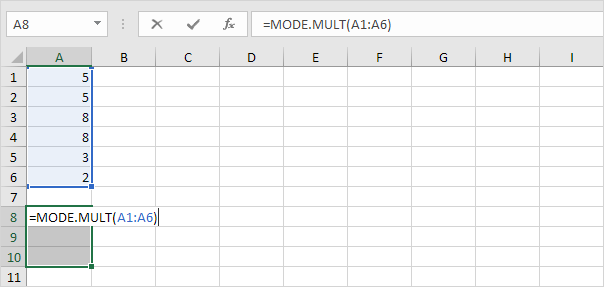7. Finish by pressing CTRL + SHIFT + ENTER.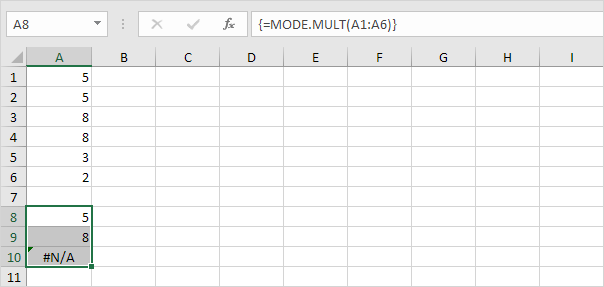Note: the formula bar indicates that this is an array formula by enclosing it in curly braces {}. To delete this array formula, select the range A8:A10 and press Delete. In this example, there are only two modes (5 and 8) so we could have selected two cells at step 5.

8. Change the value in cell A6 to 3.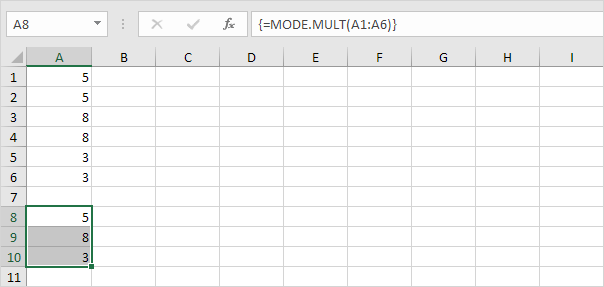9. The MODE function only works with numbers. Use INDEX, MODE and MATCH to find the most frequently occurring word in Excel.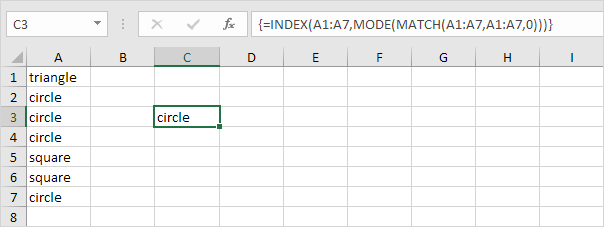Go to Next Chapter: Round